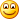## Rhino ETL Union Operation

time to read 40 min | 7916 words

Yes, it is somewhat of a blast from the past, but I just got asked how to create a good Union All operation for Rhino ETL.

The obvious implementation is:

`   1: public class UnionAllOperation : AbstractOperation`
`   2: {`
`   3:     private readonly List<IOperation> _operations = new List<IOperation>(); `
`   4:  `
`   5:     public override IEnumerable<Row> Execute(IEnumerable<Row> rows)`
`   6:     {`
`   7:         foreach (var operation in _operations)`
`   8:             foreach (var row in operation.Execute(null))`
`   9:                 yield return row;`
`  10:     }`
`  11:  `
`  12:     public UnionAllOperation Add(IOperation operation)`
`  13:     {`
`  14:         _operations.Add(operation);`
`  15:         return this;`
`  16:     }`
`  17: }`

The problem is that this does everything synchronously. The following code is a better impl, but note that this is notepad code, with all the implications of that.

`   1: public class UnionAllOperation : AbstractOperation`
`   2: {`
`   3:     private readonly List<IOperation> _operations = new List<IOperation>(); `
`   4:  `
`   5:     public override IEnumerable<Row> Execute(IEnumerable<Row> rows)`
`   6:     {`
`   7:         var blockingCollection = new BlockingCollection<Row>();`
`   8:         var tasks = _operations.Select(currentOp => Task.Factory.StartNew(() =>{`
`   9:                 foreach(var operation in currentOp.Execute(null))`
`  10:                 {`
`  11:                     blockingCollection.Add(operation);`
`  12:                 }`
`  13:                 blockingCollection.Add(null); // free the consumer thread`
`  14:             });`
`  15:  `
`  16:         Row r;`
`  17:         while(true){`
`  18:             if(tasks.All(x=>x.IsFaulted || x.IsCanceled || x.IsCompleted)) // all done`
`  19:                 break;`
`  20:             r = blockingCollection.Take();`
`  21:             if(r == null)`
`  22:                 continue;`
`  23:             yield return r;`
`  24:         }`
`  25:         while(blockingCollection.TryTake(out r)) {`
`  26:             if(r == null)`
`  27:                 continue;`
`  28:             yield return r;`
`  29:         }`
`  30:         Task.WaitAll(tasks.ToArray()); // raise any exception that were raised during execption`
`  31:     }`
`  32:  `
`  33:     public UnionAllOperation Add(IOperation operation)`
`  34:     {`
`  35:         _operations.Add(operation);`
`  36:         return this;`
`  37:     }`
`  38: }`

Usual caveats apply, notepad code, never actually run it, much less tested / debugged it.

Feel free to rip into it, though.

Dale Newman did some improvements, the most important one is to make sure that we aren’t going to evaluate the tasks several times (opps! I told ya it was notepad code), and now it looks like this:

`   1: /// <summary>`
`   2: /// Combines rows from all operations.`
`   3: /// </summary>`
`   4: public class UnionAllOperation : AbstractOperation {`
`   5:  `
`   6:     private readonly List<IOperation> _operations = new List<IOperation>();`
`   7:  `
`   8:     /// <summary>`
`   9:     /// Executes the added operations in parallel.`
`  10:     /// </summary>`
`  11:     /// <param name="rows"></param>`
`  12:     /// <returns></returns>`
`  13:     public override IEnumerable<Row> Execute(IEnumerable<Row> rows) {`
`  14:  `
`  15:         var blockingCollection = new BlockingCollection<Row>();`
`  16:  `
`  17:         Debug("Creating tasks for {0} operations.", _operations.Count);`
`  18:  `
`  19:         var tasks = _operations.Select(currentOp => Task.Factory.StartNew(() => {`
`  20:             Trace("Executing {0} operation.", currentOp.Name);`
`  21:             foreach (var row in currentOp.Execute(null)) {`
`  22:                 blockingCollection.Add(row);`
`  23:             }`
`  24:             blockingCollection.Add(null); // free the consumer thread`
`  25:         })).ToArray();`
`  26:  `
`  27:         Row r;`
`  28:         while (true) {`
`  29:             if (tasks.All(x => x.IsFaulted || x.IsCanceled || x.IsCompleted)) {`
`  30:                 Debug("All tasks have been canceled, have faulted, or have completed.");`
`  31:                 break;`
`  32:             }`
`  33:  `
`  34:             r = blockingCollection.Take();`
`  35:             if (r == null)`
`  36:                 continue;`
`  37:  `
`  38:             yield return r;`
`  39:  `
`  40:         }`
`  41:  `
`  42:         while (blockingCollection.TryTake(out r)) {`
`  43:             if (r == null)`
`  44:                 continue;`
`  45:             yield return r;`
`  46:         }`
`  47:  `
`  48:         Task.WaitAll(tasks); // raise any exception that were raised during execption`
`  49:  `
`  50:     }`
`  51:  `
`  52:     /// <summary>`
`  53:     /// Initializes this instance`
`  54:     /// </summary>`
`  55:     /// <param name="pipelineExecuter">The current pipeline executer.</param>`
`  56:     public override void PrepareForExecution(IPipelineExecuter pipelineExecuter) {`
`  57:         foreach (var operation in _operations) {`
`  58:             operation.PrepareForExecution(pipelineExecuter);`
`  59:         }`
`  60:     }`
`  61:  `
`  62:     /// <summary>`
`  63:     /// Add operation parameters`
`  64:     /// </summary>`
`  65:     /// <param name="ops">operations delimited by commas</param>`
`  66:     /// <returns></returns>`
`  67:     public UnionAllOperation Add(params IOperation[] ops) {`
`  68:         foreach (var operation in ops) {`
`  69:             _operations.Add(operation);`
`  70:         }`
`  71:         return this;`
`  72:     }`
`  73:  `
`  74:     /// <summary>`
`  75:     /// Add operations`
`  76:     /// </summary>`
`  77:     /// <param name="ops">an enumerable of operations</param>`
`  78:     /// <returns></returns>`
`  79:     public UnionAllOperation Add(IEnumerable<IOperation> ops) {`
`  80:         _operations.AddRange(ops);`
`  81:         return this;`
`  82:     }`
`  83:  `
`  84: }`